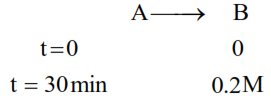# For a chemical reaction

Question:

For a chemical reaction $\mathrm{A} \rightarrow \mathrm{B}$, it was found that concentration of $\mathrm{B}$ is increased by $0.2 \mathrm{~mol} \mathrm{~L}^{-1}$ in 30 min. The average rate of the reaction is _____________$\times 10^{-1} \mathrm{~mol} \mathrm{~L}^{-1} \mathrm{~h}^{-1}$. (in nearest integer)

Solution:Av. rate of reaction $=-\frac{\Delta[\mathrm{A}]}{\Delta \mathrm{t}}=\frac{\Delta[\mathrm{B}]}{\Delta \mathrm{t}}=\frac{(0.2-0)}{\frac{1}{2}}$

$=0.4=4 \times 10^{-1} \mathrm{~mol} / \mathrm{L} \times \mathrm{hr}$